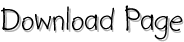## Pulse Propagation along close Conductors

#### Abstract

The propagation and reflection of arbitrarily shaped pulses on non-dispersive parallel conductors of finite length with user defined cross section is simulated employing the discretized telegraph equation. The geometry of the system of conductors and the presence of dielectric material determine the capacities and inductances that enter the calculation. The values of these parameters are found using an iterative Laplace equation solving procedure and confirmed for certain calculable geometries including the line charge inside a box. The evolving pulses and the resulting crosstalk can be plotted at any instant and - in the Mathematica notebook version of this report - be looked at in an animation. As an example a differential pair of microstrips as used in the ATLAS vertex detector is analysed.

#### Other mathematical pages:

Surface area of an ellipsoid     - a derivation of the exact formula and an approximation without elliptic functions

Discrete Fourier Transform     - a tutorial on the application of a DFT with amplitude and phase reconstruction

Infinite Products                      - a collection of infinite products and sums plus special values of elliptic theta functions

Table of integrals (elliptic functions, square roots, inverse tangents and more exotic functions), most of them not found elsewhere

 Dr. Andreas Dieckmann Physikalisches Institut der Uni Bonn Tel. 0228 732516 Raum 0.039 Nussallee 12 53115 Bonn
dieckman@physik.uni-bonn.de